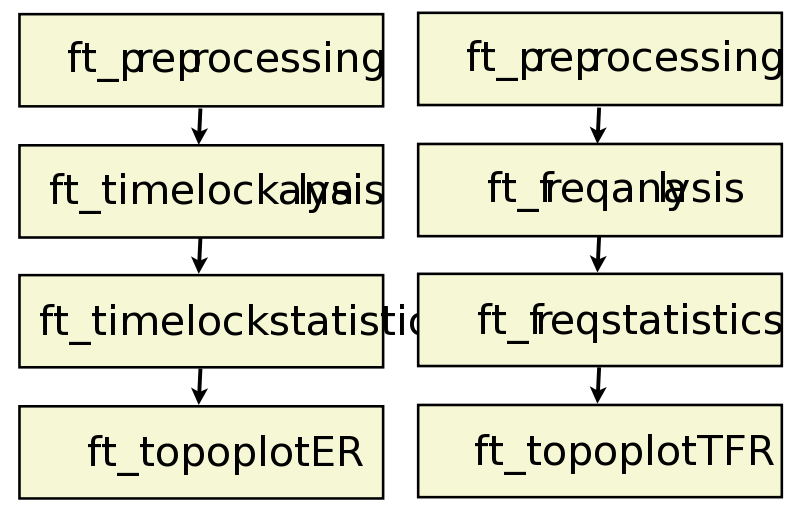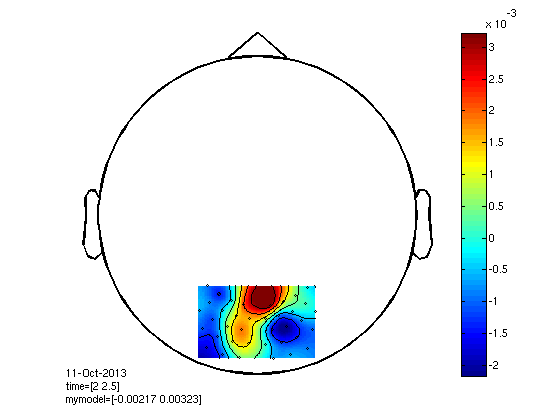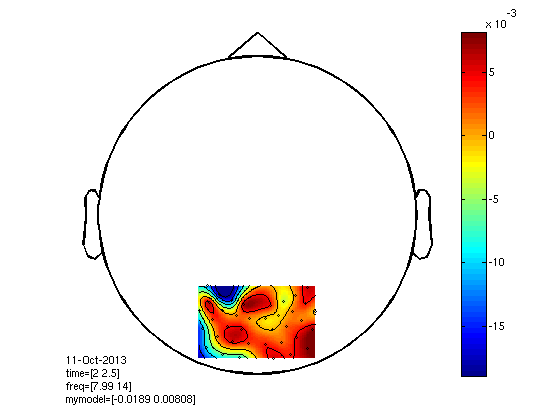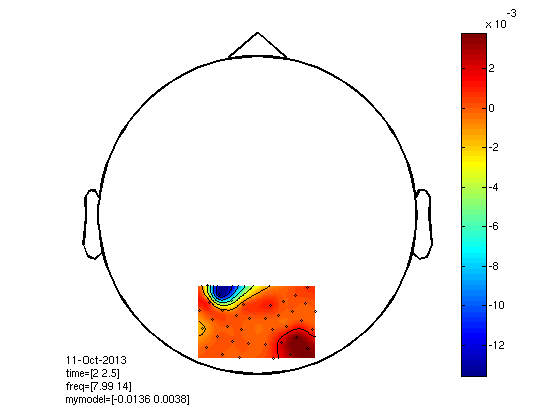# Multivariate analysis of electrophysiological data

## Introduction

The objective of this tutorial is to give an introduction to multivariate analysis of electrophysiological data. Multivariate methods aim to find task-related features in the data which allows prediction of to which task single trials belong. Note that this is very different from classical statistical testing, where such features are identified by pooling over multiple trials and/or subjects and where features are typically assumed to be independent. In this tutorial, you will learn to apply standard classification algorithms such as the support vector machine to electrophysiological data. Furthermore, you will learn about the importance of regularization. Note that FieldTrip uses the external Donders Machine Learning Toolbox (DMLT) for its multivariate analyses. This toolbox requires at least MATLAB distribution 7.6.0.324 (R2008a).

This tutorial builds on skills acquired in the preprocessing, event-related averaging and time-frequency analysis tutorials.

## Background

Multivariate methods present an alternative approach to electrophysiological data analysis. They allow statements to be made about the information content available in single trials. They also can be used for real-time analysis, which allows for new experimental designs based on the idea of brain-state dependent stimulation as well as the development of brain-computer interfaces. The methods described are also heavily used for the purpose of multivariate analysis in functional neuroimaging. For an introduction into the use of these methods please consult the following tutorial.

## Procedure

In this tutorial we will use classifiers to analyze a brain-computer interfacing dataset which has been used in this paper. In short: 275-channel MEG data was acquired while the subject was instructed with a centrally presented cue to covertly attend to the left or to the right visual hemifield (one faulty sensor was removed in the subsequent analyses). The experimental question is whether we can predict on a single-trial level to which condition (attention to the left or to the right) the single trials belong.

The data has already been segmented into the trials of interest using ft_definetrial and has been preprocessed with ft_preprocessing. The data has been detrended and downsampled to 300 Hz. The trials start at cue offset and end 2.5 seconds later. The subject has been attending to either the left or right direction during this period. No artifact rejection has been performed.

You can find the data here.

In the following, we will work our way through the time- and frequency-domain analysis pipelines as shown in the figure.### Sensor level classification in the time domain

Make sure that the multivariate toolbox at /fieldtrip_xxx/external/dmlt/ is in your MATLAB path.

``````addpath(genpath('/your-path-to-fieldtrip/external/dmlt'))
``````

We will start by analyzing the data in the time domain for our subject.

``````load covatt;
``````

We now perform a timelock analysis in order to make the data suitable as input to ft_timelockstatistics. That is, we are going to predict attention direction from temporal data. For the purpose of demonstration we will focus on occipital channels only.

``````cfg             = [];
cfg.parameter   = 'trial';
cfg.keeptrials  = 'yes';         % classifiers operate on individual trials
cfg.channel     = {'MLO' 'MRO'}; % occipital channels only
tleft   = ft_timelockanalysis(cfg, left);
tright  = ft_timelockanalysis(cfg, right);
``````

Now we specify cross-validation as a method for timelock statistics. This ensures that we will perform a classification of our data based on five-fold cross-validation. This splits up the data into five partitions or folds and attempts to build five different classifiers using the remaining four folds. The end result is then averaged over folds.

``````cfg         = [];
cfg.layout  = 'CTF275.lay';
cfg.method  = 'crossvalidate';
``````

We also need to specify a design matrix; this is simply a vector with labels 1 for the trials belonging to data for the first condition and labels 2 for trials belonging to data for the second condition

``````cfg.design  = [ones(size(tleft.trial,1),1); 2*ones(size(tright.trial,1),1)]';
``````

Let’s focus on the last segment of the data

``````cfg.latency = [2.0 2.5]; % final bit of the attention period
``````

Finally, we call ft_timelockstatistics which uses the default classification procedure; namely a standardization of the data (subtraction of the mean and division by the standard deviation), followed by applying a linear support vector machin

``````stat = ft_timelockstatistics(cfg, tleft, tright);
``````

The stat.statistic field now contains some useful statistics. By default it contains stat.accuracy (proportion of correctly classified trials) and a binomial significance test

``````stat.statistic
``````

Here, it indicates that classification performance is above chance level (0.5) and it is significant according to the binomial test (p<0.05). Note that we may be interested in other representations of classification performance such as the contingency matrix with true classes in rows and predicted classes in columns. Statistics may be specified as follow

``````cfg.statistic = {'accuracy' 'binomial' 'contingency'};
``````

when running ft_timelockstatistics. We can now, in addition, look at the contingency matri

``````stat = ft_timelockstatistics(cfg,tleft,tright);
stat.statistic.contingency
``````

We may also plot the parameters of the used classifier as if it were electrophysiological data. This is represented in the stat.model field. For each fold we have a model and each such model may contain different parameters. For example, for the default support vector machine (SVM), we have a stat.model{i}.primal field for each fold i. The easiest way to plot one of the parameters is to assign it to a different field in the stat object:

``````stat.mymodel = stat.model{1}.primal;
``````

and subsequently to treat the stat object as if it were data. The parameter field is then used to determine what to plo

``````cfg              = [];
cfg.parameter    = 'mymodel';
cfg.layout       = 'CTF275.lay';
cfg.xlim         = [2.0 2.5];
cfg.colorbar     = 'yes';
cfg.interplimits = 'electrodes';
ft_topoplotER(cfg, stat);
``````In practice, we may want to average the parameters over folds to get an average estimate of the parameters. Note further that the plot is hard to interpret. The fact that contributions extend beyond the selected channels is due to interpolation artifacts. If we look at individual features using imagesc(stat.mymodel) then it will be found that all features are used due to the way classifier operates. One way to solve this is to use dimensionality reduction or feature selection. We will see examples later in this tutorial.

#### Exercise 1

Explain which information the contingency matrix gives you, which the accuracy does not.

Redo the above analysis with a latency of [0 0.5]. Explain what you believe to be the optimal latency with which to analyze this data.

Suppose you use a dataset consisting of randomly generated data. What do you expect when you test classifier performance using the same data? And what do you expect if you use a second randomly generated dataset to test the classifier? Use the concepts of overfitting and generalization in your explanation.

Suppose you try multiple different classification procedures and find at some point that you reach a classification performance that is significantly better than chance at p=0.05. Should you trust this result? Why (not)? </div>

### Sensor level classification in the frequency domain

We now try to classify the same data in the frequency domain. Therefore, we need to perform a frequency analysis. Let’s focus on the alpha band at the end of the attention period.

``````cfg              = [];
cfg.output       = 'pow';
cfg.method       = 'mtmconvol';
cfg.taper        = 'hanning';
cfg.foi          = 8:2:14;
cfg.t_ftimwin    = ones(length(cfg.foi),1).*0.5;
cfg.channel      = {'MLO' 'MRO'};
cfg.toi          = 2.0:0.1:2.5;
cfg.keeptrials   = 'yes'; % classifiers operate on individual trials

tfrleft          = ft_freqanalysis(cfg, left);
tfrright         = ft_freqanalysis(cfg, right);
``````

Now we call freqstatistics with crossvalidate as our method.

``````cfg         = [];
cfg.layout  = 'CTF275.lay';
cfg.method  = 'crossvalidate';
cfg.design  = [ones(size(tfrleft.powspctrm,1),1); 2*ones(size(tfrright.powspctrm,1),1)]';
stat        = ft_freqstatistics(cfg,tfrleft,tfrright);
``````

We can compare classification performance with the previous results

``````stat.statistic
``````

and we see a major improvement since we are focusing on the physiologically relevant alpha band. Again, we may plot the classifier parameters to obtain a so-called importance map

``````stat.mymodel = stat.model{1}.primal;

cfg              = [];
cfg.layout       = 'CTF275.lay';
cfg.parameter    = 'mymodel';
cfg.comment      = '';
cfg.colorbar     = 'yes';
cfg.interplimits = 'electrodes';
ft_topoplotTFR(cfg, stat);
``````#### Exercise 2

Rerun the previous cross-validation with ‘cfg.nfolds=2’. Explain the difference and motivate why it is important to perform cross-validation instead of just dividing the data into one training and one test set.

### Dimensionality reduction and feature selection

One important thing to consider when classifying electrophysiological data is that we wish to reduce as much as possible the number of features used to classify the data. There are two ways to achieve this: either we map the input data to another space with a smaller number of dimensions or we select a number of dimensions from the original space. Going back to our analysis of timelocked data, we could for instance use common spatial patterns (see this paper for an explanation) to map our data to a different space. Here, instead, we perform a feature selection in the original space using a regularized classification approach. This is done by overriding the default classification procedure using the cfg.mva field:

``````cfg         = [];
cfg.layout  = 'CTF275.lay';
cfg.method  = 'crossvalidate';
cfg.design  = [ones(size(tfrleft.powspctrm,1),1); 2*ones(size(tfrright.powspctrm,1),1)]';
cfg.mva     = {dml.standardizer dml.enet('family','binomial','alpha',0.2)};
``````

This multivariate analysis standardizes the data and subsequently calls an elastic net logistic regression with regularization parameter alpha equal to 0.2. This parameter influences how many features will be selected for classification. The larger the parameter, the fewer features will be used. For a description of the used algorithm, you may consult the following paper. The elastic net algorithm gives the following result

``````stat = ft_freqstatistics(cfg, tfrleft, tfrright);
stat.statistic
``````

If we inspect stat.model{1} then we find that a different set of parameters is estimated (weights and bias). The weights are the regression coefficients of interest and bias is just an offset term. If we look at the weights then we find that just a very small number of features from the total of 912 possible features are used. This is also reflected in the topoplot.

``````stat.mymodel     = stat.model{1}.weights;

cfg              = [];
cfg.layout       = 'CTF275.lay';
cfg.parameter    = 'mymodel';
cfg.comment      = '';
cfg.colorbar     = 'yes';
cfg.interplimits = 'electrodes';
ft_topoplotTFR(cfg, stat);
``````#### Exercise 3

Use MATLAB to compute the number of non-zero elements in stat.model{1}.weights.

Why is it useful to have a representation in terms of a small number of non-zero elements?

Repeat the above analysis with a different value for alpha. Explain the results.

Alpha is a free parameter in our model. How would you determine the optimal setting for this parameter?

If we use more and more features then classification performance will first go up but eventually starts to degrade. Explain why this may happen.

Suppose we wish to select the optimal feature subset by testing all possible subsets. How many subsets do we need to test when we have n features in total?

## Conclusion

In this tutorial we have touched on a number of important issues in the classification of electrophysiological data. However, we barely scratched the surface of this field since there are many more possibilities to explore. First, many different procedures can be devised that use different forms of preprocessing, feature selection and/or prediction. For example, we may want to deal with continuous instead of discrete outputs (regression versus classification), we may want to perform a Bayesian analysis which also gives error bars on the predictions, or we may want to use timeseries analysis in order to predict changes in ongoing activity.

To use some of the more advanced methods it is required to call lower level functions. We recommend looking at the tutorials which have been written for DMLT. These can be accessed through MATLAB’s doc facility.

To construct online experimental designs that make use of multivariate analysis, for example to build BCI or neurofeedback applications, we have developed the realtime module.